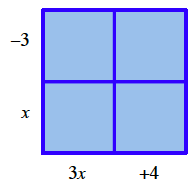### Home > CCG > Chapter 2 > Lesson 2.2.3 > Problem2-89

2-89.

Simplify the following expressions. Homework Help ✎

1. $2x+8+6x+5$

$8x+13$

2. $15+3(2x−4)−4x$

Distribute before simplifying.3. $(x−3)(3x+4)$

4. $5x(2x+7)+x(3x−5)$

Use same method as part (b).